Definitions of Square Dance Calls and ConceptsIndex -->  Plus  |  A1  |  A2  |  C1  |  C2  |  C3A  |  C3B  |  C4  |  NOL  |
Definitions (Text Only) -->  Plus  |  A1  |  A2  |  C1  |  C2  |  C3A  |  C3B  |  C4  |  NOL  |
 Find call:

(author unknown) (upphovsman okänd) (autor neznámý)

 Language: \$B8@8l(B Språk: Jazyk: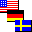All

Quadruple formations consist of four adjacent formation some of which may have positions occupied by phantoms. The given formation is usually a 4-dancer formation such as a Box, Column, Diamond, Line, or Wave. Work with the dancers and phantoms within your formation. Keep track of the phantoms and allow spaces for them upon completion of the call. When formation is a 4-dancer formation, there should be a total of 16 dancer | phantom positions before and after each call. Quadruple formations \$B\$O(B 4 \$B\$D\$NNY\$j9g\$C\$?(B formation \$B\$+\$i@.\$j(B, \$B\$=\$N\$&\$A\$N\$\$\$/\$D\$+\$K\$O(B phantom \$B\$N(B position \$B\$,\$"\$j\$^\$9(B. \$BM?\$(\$i\$l\$k(B formation \$B\$OIaDL(B, \$BNc\$(\$P(B Box, Column, Diamond, Line, \$B\$^\$?\$O(B Wave \$B\$N\$h\$&\$J(B 4 \$B?M\$N(B formation \$B\$G\$9(B. \$B<+J,\$N(B formation \$B\$G(B, \$B?M\$H(B phantom \$B\$H0l=o\$KF0\$-\$^\$9(B. Phantom \$B\$NF0\$-\$rDI\$C\$F(B, \$B%3!<%k\$N=*\$o\$j\$G\$O(B, phantom \$B\$N>l=j\$r6u\$1\$k\$h\$&\$K\$7\$^\$9(B. Formation \$B\$,(B 4 \$B?M\$N(B formation \$B\$N\$H\$-(B, \$BA4BN\$G\$O(B, \$B%3!<%k\$NA0\$H8e\$NN>J}\$H\$b(B, 16 \$B\$N?M\$^\$?\$O(B phantom \$B\$N(B position \$B\$,\$"\$j\$^\$9(B.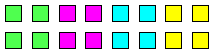Quadruple Box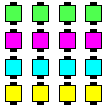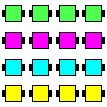Quadruple Line Quadruple Column

Notes: \$BCm Kommentarer: Poznámky:

• Quadruple formation is analogous to Triple formation except that there are 4 occurrences of the given formations instead of 3. Quadruple formation \$B\$O(B Triple formation \$B\$HF1\$8\$h\$&\$J;H\$o\$lJ}\$G\$9(B. \$B\$?\$@\$7(B, formation \$B\$N8D?t\$,(B 3 \$B\$G\$J\$/(B 4 \$B\$K\$J\$j\$^\$9(B.
• When doing a call in Quadruple formation, work only with the dancers and phantoms within your formation. Shape-changing calls are allowed and will end in a different set of Quadruple formations. Quadruple formation \$B\$G%3!<%k\$rF0\$/\$H\$-(B, \$B<+J,\$N(B formation \$B\$NCf\$@\$1\$G(B, \$B?M\$H(B phantom \$B\$HF0\$-\$^\$9(B. Shape-change \$B\$N%3!<%k\$r9T\$&\$3\$H\$,\$G\$-(B, \$B\$=\$N>l9g(B, \$B0[\$J\$k%?%\$%W\$N(B Quadruple formation \$B\$G=*\$o\$j\$^\$9(B.
• Quadruple formation is clearer for dancers than Split Phantom formation, as dancers only need to track their 4 spots, rather than the 8 spots for Split Phantom. Quadruple formation \$B\$O(B, \$B%@%s%5!<\$K\$H\$C\$F(B Split Phantom formation \$B\$h\$jJ,\$+\$j0W\$\$\$G\$9(B. Split Phantom \$B\$G\$O(B 8 \$B\$D\$N(B spot \$B\$GF0\$-\$rDI\$\$\$^\$9\$,(B, Quadruple \$B\$G\$O(B 4 \$B\$D\$N(B spot \$B\$@\$1\$K\$J\$k\$+\$i\$G\$9(B.
• Typically, a long axis traverses through the entire set of 4 formations. Visualize surrounding each formation within an imaginary bubble. The long axis is the line that traverses the 4 formations, connecting the four bubbles in a linear fashion. Dancers work within their bubble to do the given call, which may be a shape changer. Upon completion of the call, the new formation (including any phantoms) is still enclosed in a bubble shaped to enclose the new formation, and the bubbles must still be connected in a linear fashion. Stay within your formation and adjust the four bubbles as necessary to line up side-by-side along the original long axis. \$BDL>o\$O(B, 4 \$B\$D\$N(B formation \$B\$NA4BN\$r2#@Z\$kD9<4\$,\$"\$j\$^\$9(B. \$B3F!9\$N(B formation \$B\$rJq\$`2>A[IwA%\$r9M\$(\$F\$_\$F\$/\$@\$5\$\$(B. 4 \$B\$D\$NIwA%\$G\$^\$C\$9\$0\$K\$D\$J\$2\$i\$l\$?(B 4 \$B\$D\$N(B formation \$B\$r2#@Z\$k\$b\$N\$,D9<4\$G\$9(B. \$B%@%s%5!<\$OIwA%\$NCf\$G(B, \$BM?\$(\$i\$l\$?%3!<%k\$r9T\$\$\$^\$9(B. \$B\$=\$N%3!<%k\$O(B shape changer \$B\$G\$b\$+\$^\$\$\$^\$;\$s(B. \$B%3!<%k\$N=*\$o\$j\$G(B (phantom \$B\$r4^\$`(B) \$B?7\$7\$\$(B formation \$B\$O(B, \$B0MA3\$H\$7\$FIwA%\$NCf\$K\$"\$j\$^\$9(B. \$B\$=\$7\$F(B, \$BIwA%\$O0MA3\$H\$7\$F\$^\$C\$9\$0\$K\$D\$J\$,\$C\$F\$\$\$^\$9(B. \$B<+J,\$N(B formation \$B\$NCf\$K\$KN1\$^\$j(B, 4 \$B\$D\$NIwA%\$,;O\$a\$ND9<4\$K1h\$C\$FJB\$V\$h\$&\$K(B, \$B0LCV\$r(B adjust \$B\$7\$^\$9(B.
• Assume that phantoms are facing in whatever direction is needed to do the given call. For Quadruple Wave, phantoms must be facing such that each formation is a Wave. The caller can optionally be more specific and give the exact name of the formation (e.g., Quadruple R-H Two-Faced Line). Phantom \$B\$O\$I\$A\$i\$r8~\$\$\$F\$\$\$F\$bNI\$/(B, \$BM?\$(\$i\$l\$?%3!<%k\$r9T\$&\$?\$a\$K(B, \$B8~\$-\$,7h\$^\$j\$^\$9(B. Quadruple Wave \$B\$G\$"\$l\$P(B, formation \$B\$,(B wave \$B\$K\$J\$k\$h\$&\$K(B phantom \$B\$N8~\$-\$r7h\$a\$^\$9(B. \$B%3!<%i!<\$O(B, \$B\$b\$C\$H\$O\$C\$-\$j\$H(B formation \$B\$NL>A0\$r(B (\$BNc\$(\$P(B, Quadruple Right-Hand Two-Faced Line \$B\$N\$h\$&\$K(B) \$B;XDj\$9\$k\$3\$H\$,\$G\$-\$^\$9(B.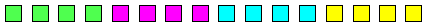(End-to-End) Quadruple Column|Line|Wave (Infrequently used) (\$B\$^\$l\$K;H\$o\$l\$^\$9(B)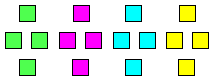Quadruple Diamond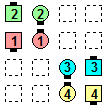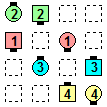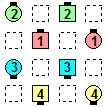before före před Quadruple Wave Swing Thru\$B\$NA0(B after efter po 1/2 by the Right\$B\$N8e(B after efter po 1/2 by the Left\$B\$N8e(B (done) (\$B=*\$o\$j(B) (klart) (hotovo)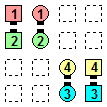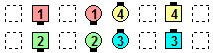before före před Quadruple Column Pass & Roll\$B\$NA0(B after efter po \$B8e(B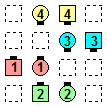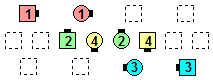before före před Quadruple Column Dixie Diamond\$B\$NA0(B after efter po \$B8e(B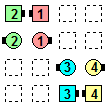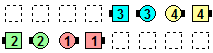before före před Quadruple Wave Turn The Key\$B\$NA0(B after efter po \$B8e(B

Note: Quadruple Wave Turn The Key is not the same as Split Phantom Waves Turn The Key. \$BCm

In the former case, dancers work within their Wave of 4, and the Counter Rotate 1/4 is a Lockit;
whereas in the latter case, the Counter Rotate 1/4 is an 8-dancer (Parallel Lines) movement.
\$BA0
\$B8e

Sometimes, the outside formations can be at a 90° angle to the inside formations. For example, \$B30B&\$N(B formation \$B\$OFbB&\$N(B formation \$B\$KBP\$7\$F(B 90° \$B\$N3QEY\$H\$J\$k\$3\$H\$,\$"\$j\$^\$9(B. \$BNc\$(\$P(B,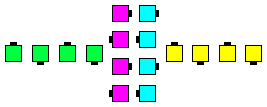Quadruple Wave

The formation may occasionally be a non-4-dancer formation. For example, Formation \$B\$O(B, \$B\$?\$^\$K\$O(B 4 \$B?M\$G\$J\$\$\$3\$H\$b\$"\$j\$^\$9(B. \$BNc\$(\$P(B,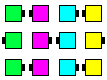Quadruple Wave of 3

Also possible, but rarely seen, are 6- or 8-dancer Quadruple formations (e.g., Quadruple Tall Six, Quadruple Hourglass, etc.). \$B\$^\$?(B, 6 \$B?M\$^\$?\$O(B 8 \$B?M\$N(B Quadruple formation (\$BNc\$(\$P(B, Quadruple Tall Six, Quadruple Hourglass, \$B\$J\$I(B) \$B\$,2DG=\$G\$9\$,(B, \$B\$[\$H\$s\$I;H\$o\$l\$^\$;\$s(B.Choreography for Quadruple formation ConceptComments? Questions? Suggestions?